Third of an hour

How many minutes is a third of an hour? Do you know to determine a third of the lesson hour (45min)?

Result

a =  20 min
b =  15 min

Solution:Leave us a comment of this math problem and its solution (i.e. if it is still somewhat unclear...):Be the first to comment!To solve this verbal math problem are needed these knowledge from mathematics:

Need help calculate sum, simplify or multiply fractions? Try our fraction calculator.

Next similar math problems:

1. Workshop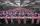In the workshop have to be produced for 5 days 2000 components. How many components must be produced in the workshop every day and how much per hour, if shift is 8 hours.
2. Cars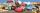Johnny has 370 cars. Peter twice less. How many cars have Peter?
3. Bakers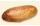Baker Martin baked 5 times more cakes than Dennis. How many cakes baked Dennis if Martin bake 25 cakes?
4. Book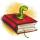Louise read from their favorite book for three days 18 pages. Every day read the same number of pages. How many pages she read every day?
5. Multiples of int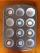How many multiples of 3 is between the numbers to 20? (zero not count)
6. Fractions 4How many 2/3s are in 6?
7. Pitcher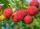Matthew picked up half-pitcher of raspberry in 45 minutes. Find how long three children filled the pitcher if each of them working at the same pace as Matthew.
8. Minutes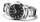Write as fraction in basic form which part of the week is 980 minutes.
9. Salary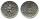Mr. Vesely got for work 874 CZK (Czech Republic Koruna). Mr. Jaros got twice less than Mr. Vesely. How much CZK got Mr. Jaros?
10. BreederSeven hens breeder has supplied 350 eggs. How long can deliver, if each of its chickens can withstand at least 5 eggs for week?
11. A koala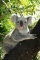A koala lives to be 14 years old in the wild. How much longer will a 2 year old koala probably live?
12. Pizza 5You have 2/4 of a pizza and you want to share it equally between 2 people how much pizza does each person get?
13. Product of two fractionsProduct of two fractions is 9 3/5 . If one of the fraction is 9 3/7. Find the other fraction.
14. Flour2 kg of flour costs 100 CZK. How much does it cost half a kilogram?
15. Dividing by five and tenNumber 5040 divide by the number 5 and by number 10: a = 5040: 5 b = 5040: 10
16. Number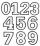Which number is 17 times larger than the number 6?
17. Fridays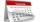Friday 13th is in 4 days. What day is today and what day it is?